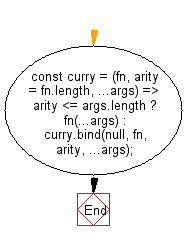# JavaScript: Curry (curries) a function

## JavaScript fundamental (ES6 Syntax): Exercise-46 with Solution

Write a JavaScript program to curry (curries) a function

According to wiki.haskell.org "Currying is the process of transforming a function that takes multiple arguments into a function that takes just a single argument and returns another function if any arguments are still needed. f x y = g (x,y), however the curried form is usually more convenient because it allows partial application.

Note: Use recursion. If the number of provided arguments (args) is sufficient, call the passed function fn. Otherwise, return a curried function fn that expects the rest of the arguments. If you want to curry a function that accepts a variable number of arguments (a variadic function, e.g. Math.min()), you can optionally pass the number of arguments to the second parameter arity.

• Use recursion.
• If the number of provided arguments (args) is sufficient, call the passed function fn.
• Otherwise, use Function.prototype.bind() to return a curried function fn that expects the rest of the arguments.
• If you want to curry a function that accepts a variable number of arguments (a variadic function, e.g. Math.min()), you can optionally pass the number of arguments to the second parameter arity.

Sample Solution:

JavaScript Code:

``````//#Source https://bit.ly/2neWfJ2
const curry = (fn, arity = fn.length, ...args) =>
arity <= args.length ? fn(...args) : curry.bind(null, fn, arity, ...args);
console.log(curry(Math.pow)(2)(8));
console.log(curry(Math.min, 3)(10)(50)(2));
```
```

Sample Output:

```256
2
```

Flowchart:Live Demo:

See the Pen javascript-basic-exercise-1-46 by w3resource (@w3resource) on CodePen.

Improve this sample solution and post your code through Disqus

What is the difficulty level of this exercise?

Test your Programming skills with w3resource's quiz.

﻿

## JavaScript: Tips of the Day

Chunks an array into n smaller arrays

Example:

```const tips_chunkIntoN = (arr, n) => {
const size = Math.ceil(arr.length / n);
return Array.from({ length: n }, (v, i) =>
arr.slice(i * size, i * size + size)
);
}
console.log(tips_chunkIntoN([1, 2, 3, 4, 5, 6, 7,8], 4));
```

Output:

```[[1,2],[3,4],[5,6],[7,8]]
```